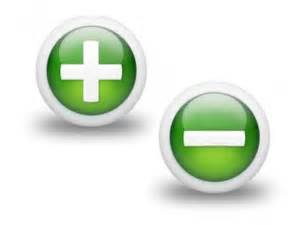# Friday Challenge – AverageIf NOT Blank or NOT Zero with Negative Values for Non-Contiguous RangesIn a  recent Friday Challenge, you were tasked with creating a formula that would work out for a non-contiguous (not connected) range that ignored Zero and Ignored Blanks.  But, what we didn’t take into account was how to modify the formula to react to negative values in our average non-contiguous range.

Here was the original challenge and some responses:

Friday Challenge – AverageIf NOT Blank or NOT Zero – NOT So Easy

Here was my solution and other responses:

How-to AverageIf Excluding Zeros and Blanks for Non-Contiguous Ranges in Excel

So the challenge is, how to modify either of these solutions for negative values.

If we enter these values, our previous solution will return 0.5 as the average when it should be 0.333

C3= Blank

E3= 0

G3= 1

I3= -10

K3= 10

Let me know how you solve adding negative values to this calculation in the comments below.

Steve=True

1.Laura

Version 1 Modification from previous challenge
Change formula to:
=AVERAGEIFS(B2:K2,\$B\$3:\$K\$3,”X”,B2:K2,”0″)
Where B2:K2 DOES NOT equal Zero
worked for me!

•SteveEqualsTrue

Hi Laura, thanks for the comment. It looks like you are using the helper row setup. However, when I test your formula, I am getting 0 as the result. I think it is because you are missing the > operator before your 0. I think you may have meant this: =AVERAGEIFS(B2:K2,\$B\$3:\$K\$3,”X”,B2:K2,”<>0″). Thanks again. Steve=Tre

•Chad R

I’m pretty sure this does it also. =SUM(C3,E3,G3,I3,K3)/INDEX(FREQUENCY((C3,E3,G3,I3,K3),0)+FREQUENCY((C2,E2,G2,I2,K2),-1),1)

•SteveEqualsTrue

Thanks Chad R. I am getting a value of 0 when I put it in. Then I noticed that you have Rows 2 as well as Rows 3 in your formula. When I change it all to the same row, then it works. Also, I was able to shorten this formula in my solution to only 1 frequency function. See if you can do it. 🙂 Thanks again. Steve=True

•SteveEqualsTrue

Chad R, I take back my comment. Looking again at my solution, yours is very Similar to mine. Thanks again. Steve=True

• # If you found the website and tutorials helpful, please consider donating to keep the lights on.

## Donate with PayPal here:•Leonid Koyfman

{=SUM(C2,E2,G2,I2,K2)/COUNT((1/CHOOSE({1,2,3,4,5},C2,E2,G2,I2,K2)))} will return 0.33

•SteveEqualsTrue

Thanks Leonid. What an awesome solution! For the readers, if you used this formula, make sure you press CTRL+SHIFT+ENTER when you input the formula as it is an a array formula. Way COOL!